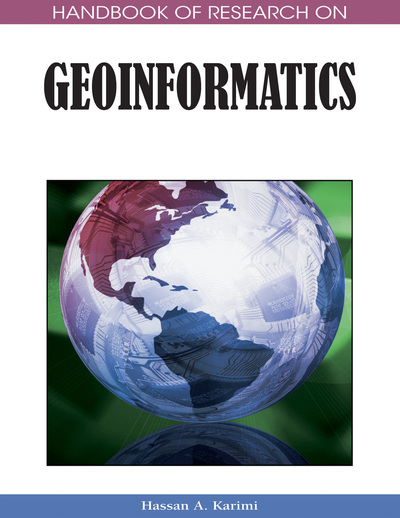# Dealing with 3D Surface Models: Raster and TIN

Mahbubur R. Meenar (Temple University, USA) and John A. Sorrentino (Temple University, USA)
Copyright: © 2009 |Pages: 9
DOI: 10.4018/978-1-59140-995-3.ch010

## Abstract

Three-dimensional surface modeling has become an important element in the processing and visualization of geographic information. Models are created from a finite sample of data points over the relevant area. The techniques used for these activities can be broadly divided into raster-based interpolation methods and vector-based triangulation methods. This chapter contains a discussion of the benefits and costs of each set of methods. The functions available using 3D surface models include elevation, queries, contours, slope and aspect, hillshade, and viewshed. Applications include modeling elevation, pollution concentration and run-off and erosion potential. The chapter ends with a brief discussion of future trends, and concludes that the choice among the methods depends on the nature of the input data and the goals of the analyst.
Chapter Preview
Top

## Introduction

A surface can be defined as a continuously variable and measurable field that contains an infinite number of points. It typically embodies a great deal of information, and may vary in elevation or proximity to a feature (Bratt, 2004, O’Sullivan et al., 2003). The points that create a 3D topographic surface store elevation or z-values on the z-axis in a three-dimensional x, y, z coordinate system. Other examples of using continuous 3D surfaces are climate, meteorology, pollution, land cover, and natural resources (McCloy, 2006, Lo et al., 2002, Schneider, 2001).

In a Geographic Information Systems (GIS) environment, a surface cannot measure or record the z-values of all of the infinite points stored inside it. A surface model, commonly known as a Digital Terrain Model (DTM), or more generally, a Digital Elevation Model (DEM), is used to create 3D surfaces in various areas of science and technology. The surface model primarily follows a two step process in recording and storing data: sampling and interpolation (O’Sullivan et al., 2003, Heywood et al., 2002). The model selects sample observation points, either systematically or adaptively; takes values of this finite number of points; interpolates the values between these points; and finally stores the output information (McCloy, 2006; Bratt, 2004, Heywood et al., 2002).

There are six alternative ways, as shown in Figure 1, to express the continuous variation of a surface in digital representation: (1) regularly spaced sample points (e.g., a 10 meter spacing DEM); (2) irregularly spaced sample points (e.g., temperature recorded at different weather stations); (3) rectangular cells (e.g., values of reflected radiation in a remotely sensed scene); (4) irregularly shaped polygons (e.g., vegetation cover class of different parcels); (5) irregular network of triangles (e.g., elevation in a Triangulated Irregular Network or TIN); and (6) polylines representing contours (e.g., elevation in contour lines) (Longley et al., 2005b).

Among all these surfaces, numbers 1 and 3 are rasters and the remaining four are vectors, which use points (number 2), lines (number 6), or polygons (numbers 4 and 5). The DEMs or DTMs may be derived from a number of data sources, including contour and spot height information, stereoscopic aerial photography or photogrammetry, satellite images, cartography (existing maps), and ground surveys (Heywood et al., 2002, Lo et al., 2002, Cohen-Or et al., 1995). Figure 2. gives an idea about different surface data collection methods and the techniques they use.

## Key Terms in this Chapter

Delaunay Triangulation: The triangulation which is equivalent to the nerve of the cells in a Voronoi diagram, i.e., that triangulation of the convex hull of the points in the diagram in which every circumcircle of a triangle is an empty circle (Okabe et al. 1992, p. 94).

Spatial Interpolation: The prediction of exact values of attributes at unsampled locations using data from sampled locations.

Digital Photogrammetry: The technology of acquiring topographic data from ortho-photos.

Raster Surface: An evenly spaced grid containing a value in each cell.

Grid: A geographic data model representing information as an array of equally sized square cells arranged in rows and columns and referenced by their geographic (x,y) locations.

Control Point: The points that provide known values in the surface creation process. Such points can be chosen randomly, located at important places, such as peaks or pits, or located as GRID samples.

TIN Surface: A surface upon which the vertices of triangles form irregularly spaced nodes which allows dense information in complex areas.

Vector Surface: The surface data that is comprised of points, lines, and polygons.

## Complete Chapter List

Search this Book:
Reset